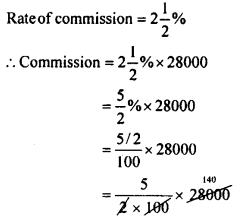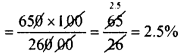# KSEEB Solutions for Class 8 Maths Chapter 9 Commercial Arithmetic Ex 9.4

Students can Download Maths Chapter 9 Commercial Arithmetic Ex 9.4 Questions and Answers, Notes Pdf, KSEEB Solutions for Class 8 Maths helps you to revise the complete Karnataka State Board Syllabus and score more marks in your examinations.

## Karnataka Board Class 8 Maths Chapter 9 Commercial Arithmetic Ex 9.4

Question 1.
Sindu sells her scooty for Rs. 28,000 through a broker. The rate of brokerage is 2%. Find the commission that the agent gets and the net amount Sindhu gets.
Price of the scooty Rs. 28,000Commission = Rs. 700
The agent gets Rs. 700
Net amount sindhu gets = 28000 – 700 = Rs. 27,300

Question 2.
A share agent sells 2000 shares at Rs. 45 each and gets the commission at the rate of 1.5%. Find the amount the agent gets.
The S.R of 1 share = Rs. 45
The S.P. of 2000 shares = 2000 × 45 = Rs. 90,000
The rate of commission = 1.5%
∴ The commission =$\frac{1.5 \times 90000}{100}$ = Rs. 1350.0
The agent gets Rs. 1350.

Question 3.
A person insures Rs. 26,000 through an insurance agent. If the agent gets Rs. 650 as the commission. find the rate of commission.
For Rs. 26,000 the commission is Rs. 650
For Rs. 100 the commissionRate of commission = 2.5%

Question 4.
A selling agent gets Rs. 10,200 in a month. This includes his monthly salary of Rs. 6000 and 6% commission for the sales. Find the value of the goods he sold.$\frac{100 \times 4200}{6}$